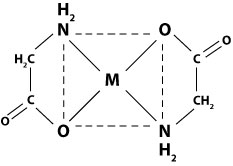# Crystal Field Splitting (Worksheets)

Name: ______________________________

Section: _____________________________

Student ID#:__________________________

Work in groups on these problems. You should try to answer the questions without referring to your textbook. If you get stuck, try asking another group for help.

## Q1

Calculate the Crystal Field Stabilization Energy for both high spin and low spin octahedral complexes of $$Co(gly)_6^{3-}$$. Which is preferred?## Q2

Determine whether the $$Co^{+2}$$ complex with phenanthroline will prefer to be octahedral or tetrahedral based on Crystal Field Stabilization Energy.

## Q3

Draw the M.O. diagram for an octahedral complex with six sigma donor ligands. Draw the electrons for a $$d^5$$ high spin complex.

## Q4

What are Jahn-Teller distortions? Where do they come from? Show 'z - in' and 'z-out' configurations. Which is preferred for a $$d^9$$ configuration?

## Q5

Draw the expected splitting for a $$Cu(ox)_3^{4-}$$ complex. Would the splitting pattern change if two of the Cu-O bond lengths were longer than the other four?

## Q6

Using the Tanabe-Sugano diagrams, give the ground states for the free metal, high spin and low spin $$d^5$$ complexes. What are the first excited state for each of these? List all possible transitions. Draw the microstates of the ground state for each of the three species.

## Q7

Determine the spin state for each of the following:

• $$Fe(OH_2)_6^{3+}$$
• $$V(CN)_6^{3-}$$
• $$CuI_4^{2-}$$
• $$RuCl_6^{4-}$$

## Q8

Draw the expected splitting of the d orbitals for a trigonal bipyramidal geometry

## Q9

Draw the high spin and low spin configurations for a $$d^4$$ ion in a tetrahedral field. Which is preferred and why?

## Q10

Determine the Crystal Field Stabilization Energy for the tetrahedral $$Co^{+2}$$ complex with methylamine.